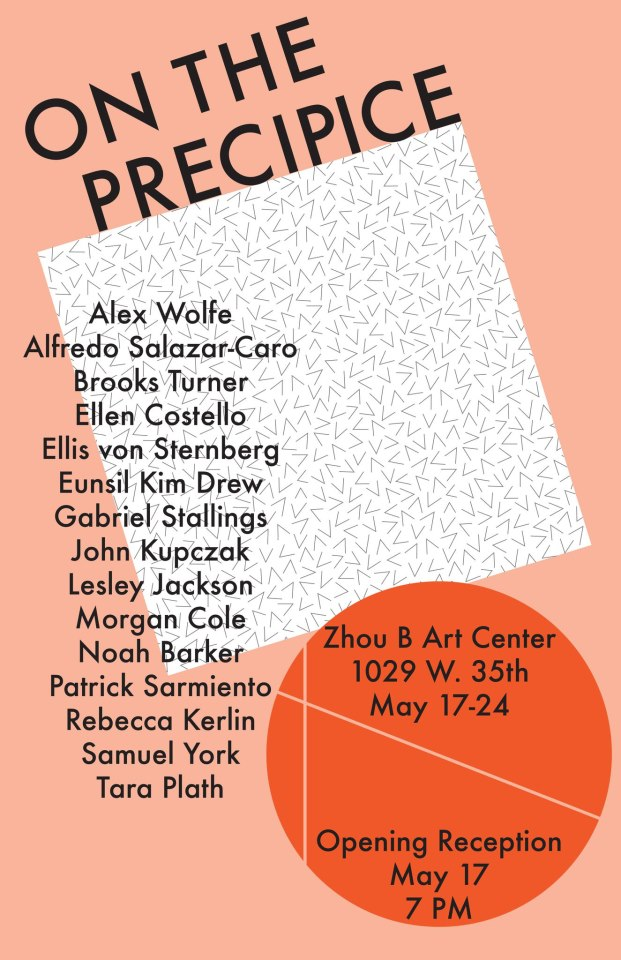May 17th 2013

# On The Precipice

## On view through Friday, May 24th

On The Precipice

Behold the precipice. The edge. The inevitable.
Come celebrate the leap onto the void of reality.

featuring works by:

A l e x W o l f e
A l f r e d o S a l a z a r – C a r o
B r o o k s T u r n e r
E l l e n C o s t e l l o
E l l i s v o n S t e r n b e r g
E u n s i l K i m D r e w
G a b r i e l S t a l l i n g s
J o h n K u p c z a k
L e s l e y J a c k s o n
M o r g a n C o l e
N o a h B a r k e r
P a t r i c k S a r m i e n t o
R e b e c c a K e r l i n
S a m u e l Y o r k
T a r a P l a t h

### Tags: Bridgeport, ZhouBArtCenter